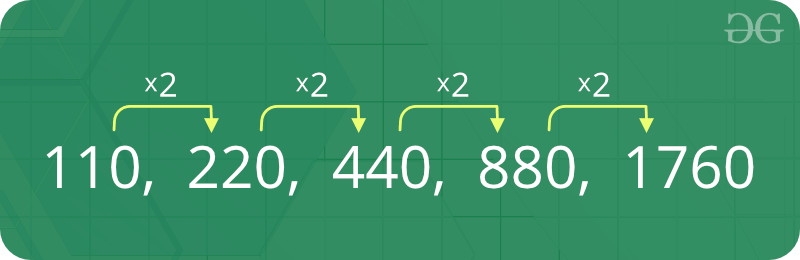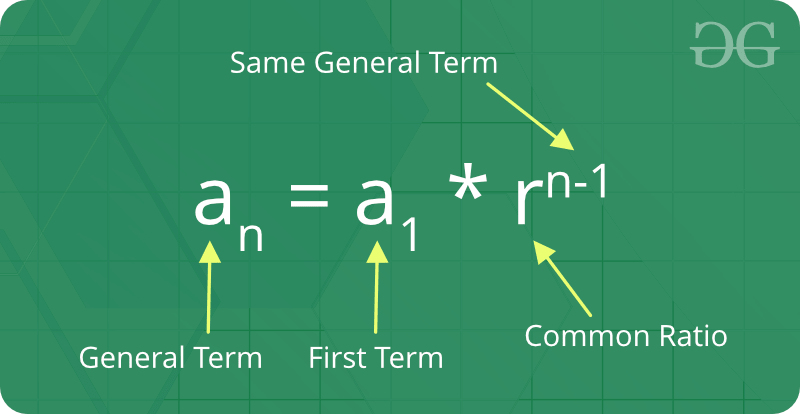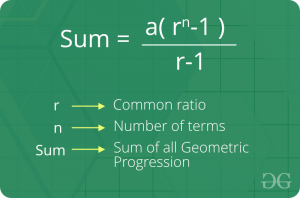# Geometric Progression

• Last Updated : 06 Jun, 2021

A sequence of numbers is called a Geometric progression if the ratio of any two consecutive terms is always same. In simple terms, it means that next number in the series is calculated by multiplying a fixed number to the previous number in the series.For example, 2, 4, 8, 16 is a GP because ratio of any two consecutive terms in the series (common difference) is same (4 / 2 = 8 / 4 = 16 / 8 = 2).Attention reader! Don’t stop learning now. Get hold of all the important mathematical concepts for competitive programming with the Essential Maths for CP Course at a student-friendly price. To complete your preparation from learning a language to DS Algo and many more,  please refer Complete Interview Preparation Course.

1. Initial term: In a geometric progression, the first number is called the initial term.
2. Common ratio: The ratio between a term in the sequence and the term before it is called the “common ratio.”
3. The behaviour of a geometric sequence depends on the value of the common ratio. If the common ratio is:
• Positive, the terms will all be the same sign as the initial term.
• Negative, the terms will alternate between positive and negative.
• Greater than 1, there will be exponential growth towards positive or negative infinity (depending on the sign of the initial term).
• 1, the progression is a constant sequence.
• Between -1 and 1 but not zero, there will be exponential decay towards zero.
• -1, the progression is an alternating sequence.
• Less than -1, for the absolute values there is exponential growth towards (unsigned) infinity, due to the alternating sign.

Formula of nth term of an Geometric Progression :
If ‘a’ is the first term and ‘r’ is the common ratio.Thus, the explicit formula isFormula of sum of nth term of Geometric Progression:How we check whether a series is geometric progression or not?
Let’s find the ratio of the consecutive terms, so we can say that the ratio of the consecutive terms of given sequences is 13 or a constant. So this sequence is forming a geometrical progression.

## C++

 `// C++ program to check if a given array``// can form geometric progression``#include ``using` `namespace` `std;` `bool` `is_geometric(``int` `arr[], ``int` `n)``{``    ``if` `(n == 1)``        ``return` `true``;` `    ``// Calculate ratio``    ``int` `ratio = arr / (arr);` `    ``// Check the ratio of the remaining``    ``for` `(``int` `i = 1; i < n; i++) {``        ``if` `((arr[i] / (arr[i - 1])) != ratio) {``            ``return` `false``;``        ``}``    ``}``    ``return` `true``;``}` `// Driven Program``int` `main()``{``    ``int` `arr[] = { 2, 6, 18, 54 };``    ``int` `n = ``sizeof``(arr) / ``sizeof``(arr);` `    ``(is_geometric(arr, n)) ? (cout << ``"True"` `<< endl) : (cout << ``"False"` `<< endl);` `    ``return` `0;``}`

## Java

 `// Java program to check if a given array``// can form geometric progression``import` `java.util.Arrays;` `class` `GFG {` `    ``// function to check series is``    ``// geometric progression or not``    ``static` `boolean` `is_geometric(``int` `arr[], ``int` `n)``    ``{``        ``if` `(n == ``1``)``            ``return` `true``;` `        ``// Calculate ratio``        ``int` `ratio = arr[``1``] / (arr[``0``]);` `        ``// Check the ratio of the remaining``        ``for` `(``int` `i = ``1``; i < n; i++) {``            ``if` `((arr[i] / (arr[i - ``1``])) != ratio) {``                ``return` `false``;``            ``}``        ``}``        ``return` `true``;``    ``}` `    ``// driver code``    ``public` `static` `void` `main(String[] args)``    ``{``        ``int` `arr[] = { ``2``, ``6``, ``18``, ``54` `};``        ``int` `n = arr.length;` `        ``if` `(is_geometric(arr, n))``            ``System.out.println(``"True"``);``        ``else``            ``System.out.println(``"False"``);``    ``}``}`

## Python3

 `def` `is_geometric(li):``    ``if` `len``(li) <``=` `1``:``        ``return` `True``        ` `    ``# Calculate ratio``    ``ratio ``=` `li[``1``]``/``float``(li[``0``])``    ` `    ``# Check the ratio of the remaining``    ``for` `i ``in` `range``(``1``, ``len``(li)):``        ``if` `li[i]``/``float``(li[i``-``1``]) !``=` `ratio:``            ``return` `False``    ``return` `True` `print``(is_geometric([``2``, ``6``, ``18``, ``54``]))`

## C#

 `// C# program to check if a given array``// can form geometric progression``using` `System;` `class` `Geeks {` `    ``static` `bool` `is_geometric(``int``[] arr, ``int` `n)``    ``{``        ``if` `(n == 1)``            ``return` `true``;` `        ``// Calculate ratio``        ``int` `ratio = arr / (arr);` `        ``// Check the ratio of the remaining``        ``for` `(``int` `i = 1; i < n; i++) {``            ``if` `((arr[i] / (arr[i - 1])) != ratio) {``                ``return` `false``;``            ``}``        ``}``        ``return` `true``;``    ``}` `    ``// Driven Program``    ``public` `static` `void` `Main(String[] args)``    ``{``        ``int``[] arr = ``new` `int``[] { 2, 6, 18, 54 };``        ``int` `n = arr.Length;` `        ``if` `(is_geometric(arr, n))``            ``Console.WriteLine(``"True"``);``        ``else``            ``Console.WriteLine(``"False"``);``    ``}``}`

## PHP

 ``

## Javascript

 ``

Output:

`True`

Basic Program related to Geometric Progression

More problems related to Geometric Progression

My Personal Notes arrow_drop_up